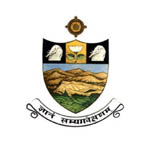# AP ICET 2020 Syllabus – Check Complete Subject Wise SyllabusAP ICET 2020 Syllabus includes the complete syllabus for both (MBA & MCA) postgraduate level entrance exam. Andhra Pradesh Integrated Common Entrance Test (AP ICET) is a state level entrance exam. Through this exam, candidates will get admission in the state universities and institutions of AP state. AP ICET will be organized by the Sri Venkateswara University, Tirupati on the behalf of APSCHE, Hyderabad. The admissions will be offered in MBA and MCA master level courses. Through this article, candidates must check complete AP ICET syllabus 2020.

## AP ICET 2020 Syllabus

AP ICET syllabus will contain the subjects such as Analytical Ability, Mathematical Ability and Communication Ability. With the help of given below syllabus, candidates must check the subjects which will be going to ask in the examination.

## Analytical Ability

In analytical ability section question will be asked from Data Sufficiency, Problem Solving etc.

### Data Sufficiency:

A question is given followed by data in the form of two statements labelled as (i) and (ii ).

Applications Closing Soon:

If the data given in (i) alone is sufficient to answer the question then choice (1) is the correct answer.

If the data given in (ii) alone is sufficient to answer the question then choice (2) is the correct answer.

If both (i) and (ii) put together are sufficient to answer the question but neither statement alone is sufficient, then choice (3) is the correct answer.

If both (i) and (ii) put together are not sufficient to answer the question and additional data is needed, then choice (4) is the correct answer.

### Problem Solving:

• Sequences and Series:Analogies of numbers and alphabet, completion of blank spaces following the pattern in a: b :: c:d relationship; odd thing out: missing number in a sequence or a series.
• Data Analysis:The data given in a Table, Graph, bar diagram, Pie Chart, Venn diagram or a Passage is to be analyzed and the questions pertaining to the data are to be answered.
• Coding and Decoding Problems:A code pattern of English Alphabet is given. A given word or a group of letters are to be coded or decoded based on the given code or codes.
• Date, Time & Arrangement Problems:Calendar problems, clock problems, blood relationships, arrivals, departures and schedules, seating arrangements, symbol and notation interpretation.

Also Check:

AP ICET Exam Pattern

AP ICET Preparation Tips

AP ICET Hall Ticket 2020

## Mathematical Ability

### Arithmetical Ability:

Laws of indices, ratio and proportion; surds; numbers and divisibility, l.c.m. and g.c.d; Rational numbers, Ordering.; Percentages; Profit and loss; Partnership, Pipes and cisterns, time, distance and work problems, areas and volumes, mensuration, modular arithmetic.

### Algebraical and Geometrical Ability:

Statements, Truth tables, implication converse and inverse, Tautologies-Sets, Relations and functions, Equation of a line in different forms, Polynomials, Remainder theorem and consequences, Linear equations and expressions, Progressions, Binomial Theorem, Matrices, Notion of a limit and derivative, Plane geometry-lines, Triangles, Quadrilaterals, Circles, Coordinate geometry-distance between points, Trigonometry – Trigonometric ratios, Trigonometric ratios of standard angles, (0°, 30°, 45°, 60°, 90°, 180°): Trigonometric identities: sample problems on heights and distances,

### Statistical Ability:

Frequency distributions, Mean, Median, Mode, Standard Deviation, Correlation, simple problems on Probability.

## Communication Ability

Candidates will be assessed on the ability to:

• Identify vocabulary used in day-to-day communication.
• Understand the functional use of grammar in day-to-day communication as well as in business contexts.
• Identify the basic terminology and concepts in computer and business contexts (letters, reports, memoranda, agenda, minutes etc.).
• Understand written text and drawing inferences.
• Part 1: Vocabulary
• Part 2: Business and Computer terminology
• Part 3: Functional Grammar
• Part 4: Reading Comprehension (3 Passages)

If you have any other queries about AP ICET 2020 Syllabus, you can leave your comments below in the comment box.Chandigarh University - 2020 Application Closing Soon

×